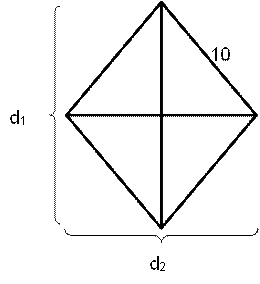# CAT 2021 Question Paper | Quant Slot 3

###### CAT Previous Year Paper | CAT Quant Questions | Question 12

CAT 2021 Quant was dominated by Arithmetic followed by Algebra. In Arithmetic, the questions were dominated by topics like Speed-time-distance, Mixture and Alligations. This year, there was a surprise. The questions from Geometry were relatively on the lower side as compared to the previous years. There were 8 TITA Qs this year. Overall this section was at a medium level of difficulty.

Question 12 : A park is shaped like a rhombus and has area 96 sq m. If 40 m of fencing is needed to enclose the park, the cost, in INR, of laying electric wires along its two diagonals, at the rate of ₹125 per m, is

## Best CAT Coaching in Chennai

#### CAT Coaching in Chennai - CAT 2022Limited Seats Available - Register Now!

The are of the rhombus is given by,
Area = ½ × d1 × d2
Where d1 & d2 are the diagonals of the rhombus.Area = ½ × d1 × d2
96 = ½ × d1 × d2
96 × 4 = 2 × d1 × d2
Also,
$$left$ $frac { d _ { 1 } } { 2 } $right$ ^ { 2 } + $left$ $frac { d _ { 2 } } { 2 } \right$ ^ { 2 } = 10 ^ { 2 }$ d12 + d22 = 400$d1 + d2 )2 = d12 + d22 + 2 × d1 × d2
(d1 + d2 )2 = 400 + 4(96)
(d1 + d2 )2 = 4(100 + 96)
(d1 + d2 )2 = 4(196)
(d1 + d2) = 2(14)
d1 + d2 = 28
The cost of laying electric wires along the diagonals at the rate of ₹125 per meter
= 28 × 125
= ₹3500

###### CAT Coaching in ChennaiCAT 2023

Classroom Batches Starting Now! @Gopalapuram

###### Best CAT Coaching in Chennai Introductory offer of 5000/-

Attend a Demo Class

##### Where is 2IIM located?

2IIM Online CAT Coaching
A Fermat Education Initiative,
58/16, Indira Gandhi Street,
Kaveri Rangan Nagar, Saligramam, Chennai 600 093

##### How to reach 2IIM?

Mobile: (91) 99626 48484 / 94459 38484
WhatsApp: WhatsApp Now
Email: info@2iim.com